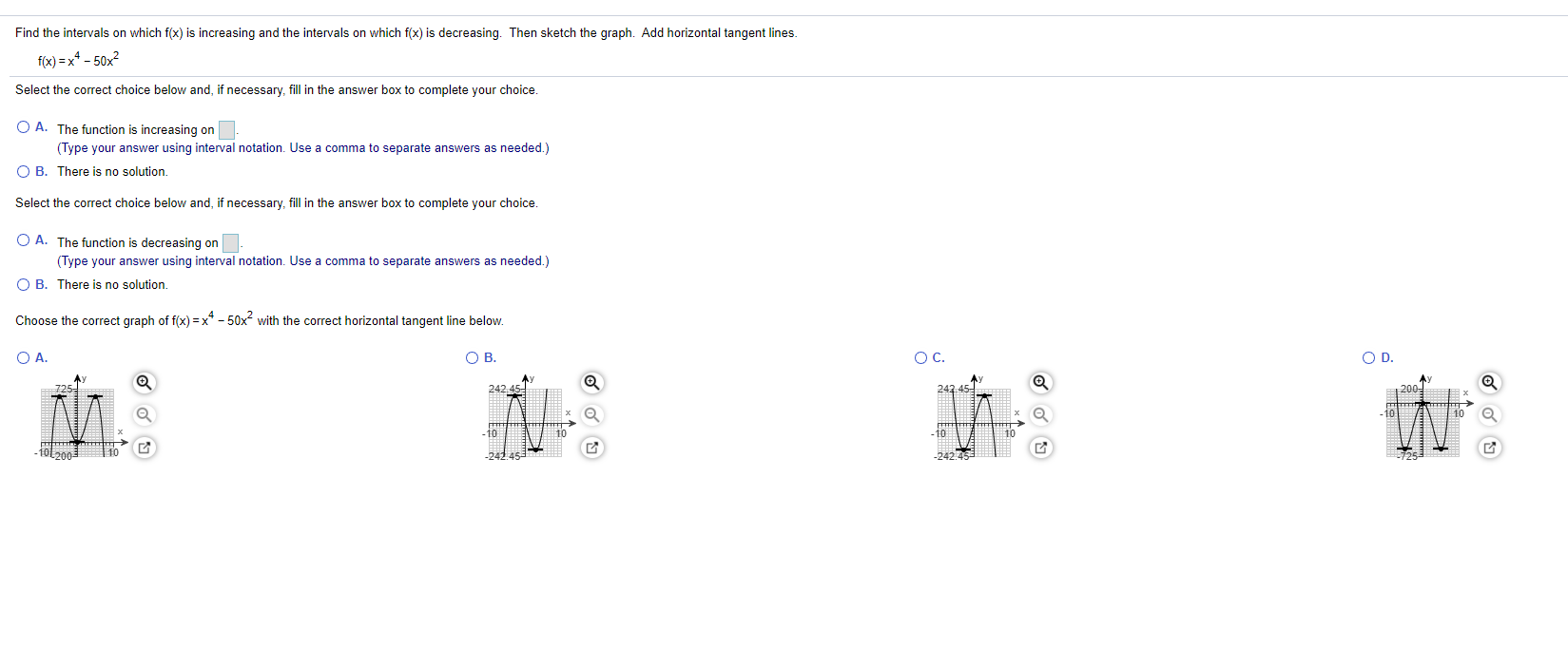### Create an Account

Already have account?

### Forgot Your Password ?

Home / Questions / Find the intervals on which f(x) is increasing and the intervals on which f(x) is decreasi...

# Find the intervals on which f(x) is increasing and the intervals on which f(x) is decreasing. Then sketch the graph. Add horizontal tangent lines. f(x)=x4 - 50x Select the correct choice below and, if

Find the intervals on which f(x) is increasing and the intervals on which f(x) is decreasing. Then sketch the graph. Add horizontal tangent lines. f(x)=x4 - 50x Select the correct choice below and, if necessary, fill in the answer box to complete your choice. O A. The function is increasing on (Type your answer using interval notation. Use a comma to separate answers as needed.) OB. There is no solution. Select the correct choice below and, if necessary, fill in the answer box to complete your choice. O A. The function is decreasing on (Type your answer using interval notation. Use a comma to separate answers as needed.) OB. There is no solution. Choose the correct graph of f(x) = x4 - 50x2 with the correct horizontal tangent line below. OA OB. ос. OD 242.45 242.45 U * KE mul D 10 -241458 -2421Apr 14 2021 View more View Less

#### Answer (Solved)Subscribe To Get Solution